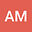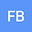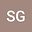Analytical representations of the Residence Time Distribution (RTD) associated with Hyporheic Exchange beneath Dune-like bedforms at different sediment bed depths
•••Fulvio Boano
Politecnico di Torino
Author ProfileStanley Grant
Virginia Tech
Author Profile## Abstract

The hyporheic exchange below dune-shaped bedforms has a great impact on the stream environment. One of the most important properties of the hyporheic zone is the residence time distribution (RTD) of flow paths in the sediment domain. Here we evaluate the influence of an impervious layer, at a dimensionless sediment depth of $$d_{b}^{*}=\frac{2\pi d_{b}}{\lambda}$$ where $$\lambda$$ is the dune wavelength, on the form of the hyporheic exchange RTD. Empirical RTDs were generated, over a range of $$d_{b}^{*\ }\$$values, from numerical particle tracking experiments in which $$10000$$ particles sinusoidally distributed over a flatbed domain were released. These empirical RTDs are best represented by the Gamma, Log-Normal and Fréchet distributions over normalized bed depth of $${0\ <=d}_{b}^{*\ }\leq 1.2$$,$${1.2<d}_{b}^{*\ }\leq 3.1$$, and $$d_{b}^{*\ }>3.1$$, respectively. The depth dependence of the analytical distribution parameters is also presented, together with a set of regression formulae to predict these parameters based on $$d_{b}^{*\ }$$with a high degree of accuracy ($$R^{2}>99.8\%$$). These results contribute to our understanding of the physical and mixing processes underpinning hyporheic exchange in streams and allow for a quick evaluation of its likely impact on nutrient and contaminant processing (e.g., based on the magnitude of the Damköhler number).
Keywords: Dunes, bedforms, residence times distribution, sediment depth effect, Hyporheic residence times, analytical representation, two parametric distributions, Damköhler Number.